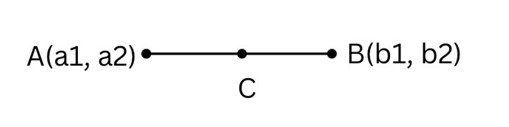# Swift Program to Find the Mid-point of a Line

This tutorial will discuss how to write swift program to find the mid-point of a line.

A point lies between the middle of two points is known as mid-point. Suppose we have two points A and B and C point is the mid-point located between A and B. Midpoint always divide lines segment in equal parts. Or we can say mid-point is always equidistant from other two points.## Formula

Following is the formula −

Midpoint = ((a1+a2)/2, (b1+b2)/2)


Below is a demonstration of the same −

Input

Suppose our given input is −

Point 1: (5.0,6.0)
Point 2: (7.0,8.0)


Output

The desired output would be -

The mid-point is: (5.5, 7.5)


## Algorithm

Following is the algorithm −

Step 1- Create a function with return value.

Step 2- Calculate mid-point using the following formula −

let res1 = (a1+a2)/2
let res2 = (b1+b2)/2


Step 3- Calling the function and pass two points in the function as a parameter.

Step 4- Print the output.

## Example

The following program shows how to calculate mid-point of a line.

import Foundation
import Glibc

// Creating a function to calculate mid-point of a line
func MidPoint(a1: Double, a2: Double, b1: Double, b2: Double){
let res1 = (a1+a2)/2
let res2 = (b1+b2)/2
print("The mid-point is: (\(res1), \(res2))")
}

// Points
var m1 = 1.0
var m2 = 2.0
var n1 = 3.0
var n2 = 4.0
print("Point 1: (\(m1),\(m2))")
print("Point 2: (\(n1),\(n2))")

// Calling function
MidPoint(a1:m1, a2:m2, b1:n1, b2:n2)


## Output

Point 1: (1.0,2.0)
Point 2: (3.0,4.0)
The mid-point is: (1.5, 3.5)


Here, in the above program we create a function which return the mid point of two points using the following formula −

let res1 = (a1+a2)/2
let res2 = (b1+b2)/2


Here we enter two points (1, 2) and (3, 4), hence the mid point is (1.5, 3.5).